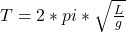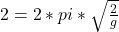## A simple pendulum is used to determine the acceleration due to gravity at the surface of a planet The pendulum has a length of 2 m and its

Question

A simple pendulum is used to determine the acceleration due to gravity at the surface of a planet The pendulum has a length of 2 m and its period is measured to be 2 s. The value of g obtained in this investigation is most nearly :A. 1 m/s2 B. 2 m/s2C. 5 m/s2 D. 10 m/s2 E. 20 m/s2

in progress 0
5 months 2021-09-05T03:59:54+00:00 1 Answers 6 views 0

## Answers ( )

E. 20 m/s^2

Explanation:

The period of a pendulum is given by the following equation:Where T = time period (in seconds)

L = length of pendulum

and g = acceleration due to gravity

Substituting the values from the question we get:Solving for g, we get:

g = 19.74 m/s^2

Closest option to this is option (E).